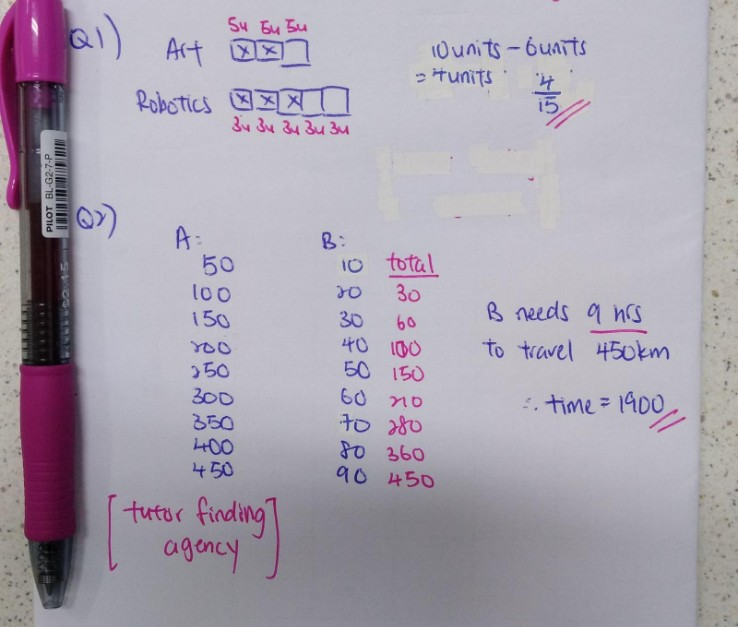# Question

Q1:

2/3 of the pupils in Class A are in the Art Club and 3/5 of the pupils from the same class are in Robotics club. Given that every pupil joins at least one of the 2 clubs, what fraction of the pupils in class A join both clubs ?

Q2:

Ali and Brenard both left Town A at 10 00. Ali covered 50km every hour. Brenard covered 10km in the first hour, 20 km in the second hour, 30 km in the third hour and so on. At what time will Bernard catchup with Ali ?0 Replies 1 Like ✔Accepted Answer

Q1. 2/3 + 3/5 = 19/15

19/15 – 1 = 4/15

Students in both clubs = 4/15

Q2. The distance between Ali and Bernard after every hour is shown below.

1. 50-10=40
2. 40+30=70
3. 70+20=90
4. 90+10=100
5. 100+0=100
6. 100-10=90
7. 90-20=70
8. 70-30=40
9. 40-40 =0

It takes 9 hours to catch up.

1000 + 9 h = 1900

0 Replies 0 Likes